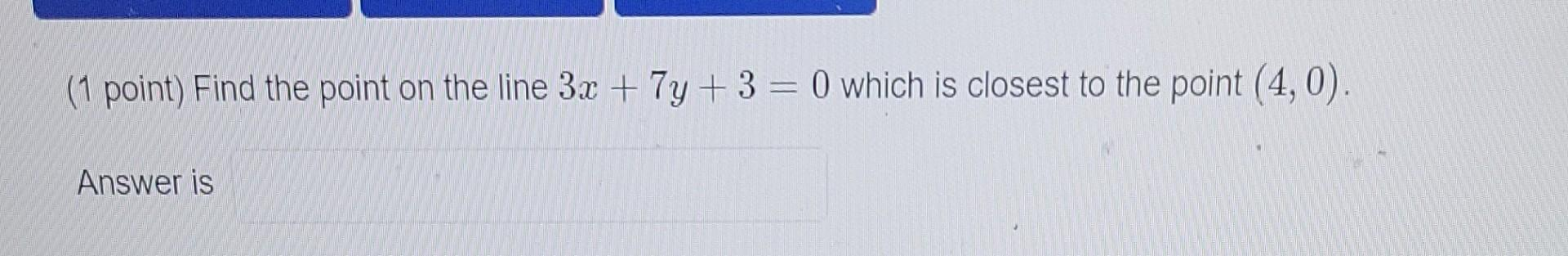# Question Can you please explain how to solve this problem Thank You. (1 point) Find the point on the line 3x + 7y + 3 = 0 which is closest to the point (4,0). Answer isCan you please explain how to solve this problem

Thank You.

Transcribed Image Text: (1 point) Find the point on the line 3x + 7y + 3 = 0 which is closest to the point (4,0). Answer is
More
Transcribed Image Text: (1 point) Find the point on the line 3x + 7y + 3 = 0 which is closest to the point (4,0). Answer is